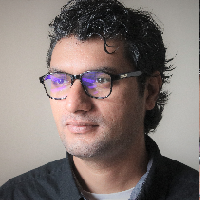# Image Classification with CNNs using Keras

In this course, we will create a Convolutional Neural Network model, which will be trained on trained on the Fashion MNIST dataset to classify images of articles of clothing in one of the 10 classes in the dataset. Convolutional Neural Network are a class of deep neural networks, most commonly applied to analysing images. In this course, we will be creating the CNN model from scratch and will not be using any pre-trained model like the ResNet50 model.Duration (mins)

Learners

#### 5.0 / 5

Rating

We will cover the following tasks in 43 minutes:

### Importing Libraries

We will understand the Rhyme interface and our learning environment. You will get a virtual machine, you will need Jupyter Notebook and TensorFlow for this course and both of these are already installed on your virtual machine. Jupyter Notebooks are very popular with Data Science and Machine Learning Engineers as one can write code in cells and use other cells for documentation. Computer vision is a complicated subject but the primary aim is to help machines gain a high-level understanding derived from images and videos. Like the name implies, computer vision is simply helping computer achieve a human-like visual system.

### Pre-process Data

We will import TensorFlow and its implementation of Keras - a high-level API to build and train deep learning models. We will also import NumPy - which is the fundamental package for scientific computing in python. We will import the Sequential class from Keras as well as many of the layers that we will be using to build our convolutional neural network model.

### Visualize Examples

We are going to use the Fashion MNIST dataset for this course. This dataset actually comes with Keras so it’s really simple to use it if you’re using Keras to build your model, which we are. This dataset has 60k training examples and 10k examples for the test set. The labels are numeric values from 0 to 9 for the 10 classes that this dataset has. The Fashion MNIST dataset has grayscale images of different type of clothing items. We will take a look at the images in a minute. The images are grayscale so there is no channel information and all the images are of 28 by 28 pixels with each pixel having a value between 0 to 255.

### Create Model

The labels in our dataset are just integers from 0 to 9. We need to transform the labels in a way that each column corresponds to each class. So, if a label has value 3, this means that the new transformed labels array will have 0s for all the columns except for column at index 3 which will have a value of 1. This type of transformation is called one hot encoding and fortunately for us, Keras provides a simple way for achieving this with the `to_categorical` method.

We will also normalize the training and test data. This is easy to achieve. We already know the maximum value a pixel can have is 255 so all we have to do is divide our examples by 255. We will also expand the dimensions on our training and test examples. This is because our images are grayscale so in the dataset there is no information provided for channels because for a grayscale image we don’t need that information. But the convolutional layers, the ones we are going to use in our model, do expect the channels to be present even if the value for channels is just 1. So, we will use NumPy’s expand dimension method to add channel information.

### Train the Model

We will use the Sequential class we loaded earlier. We can simply pass in a list of layers to the initializer. The first two layers are convolutional 2D layer. Then, we will flatten the convolutional layers so that the 4D tensors are converted to 2D tensors. The flattened output goes to a fully connected layer. We will use the Dense class here. And finally, for the output, we will need a Dense layer with the `softmax` activation function and number of classes for number of nodes.

### Final Predictions

We will compile this model with a variant of stochastic gradient descent algorithm which is called `adaptive moment estimation` or `adam` in short. We will use the loss function called `categorical cross entropy` and we will use accuracy as our training metric.

## Watch Preview

Preview the instructions that you will follow along in a hands-on session in your browser.I am a machine learning engineer with focus in computer vision and sequence modelling for automated signal processing using deep learning techniques. My previous experiences include leading chatbot development for a large corporation.

##### How is this different from YouTube, PluralSight, Udemy, etc.?
In Rhyme, all projects are completely hands-on. You don't just passively watch someone else. You use the software directly while following the host's (Amit Yadav) instructions. Using the software is the only way to achieve mastery. With the "Live Guide" option, you can ask for help and get immediate response.
##### Is this session really free?
Absolutely! Your host (Amit Yadav) has provided this session completely free of cost!